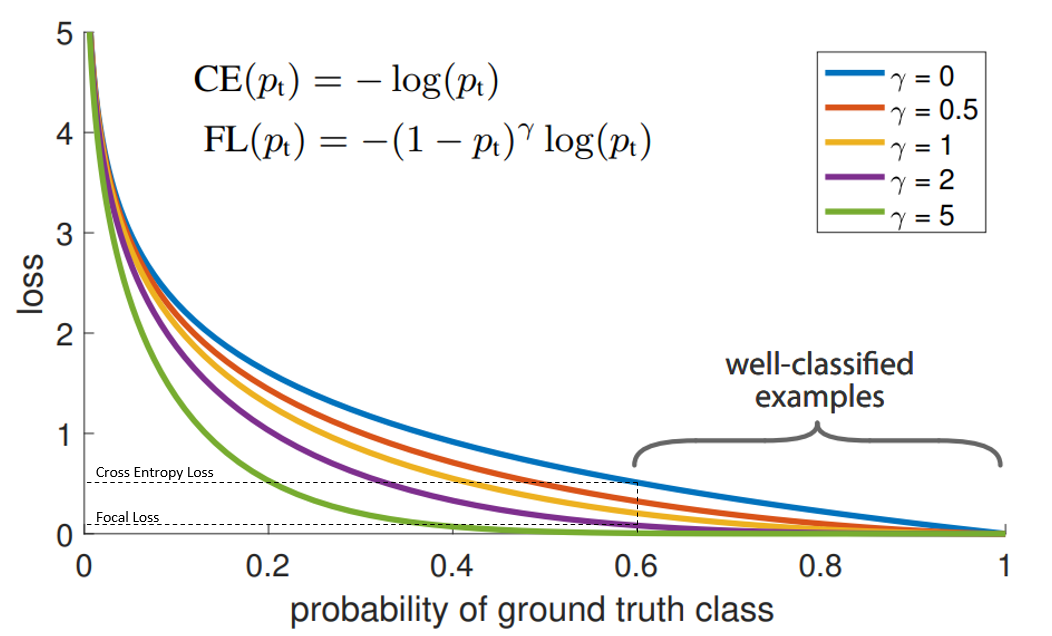# Learnings from building a classifier

• Oversampling
• Undesampling
• Weighted loss to handle imbalanceEasy samples would contribute less to the learning.(Tail of the above curve)
• We are only training 20% of the parameters, so less time take taken for training
• We can have stack of prefix tuned models(solving the same task, or diff task), while base model remains same.
• As we are not tampering the knowledge of pre-trained model(remember catastrophic fore-getting), It would be more generalisable.
• Accuracy is almost equivalent to full fine-tuning
• Dropout
• Multi Dropout
• Layer wise learning rate decay.
• Grouped learning rate decay.
• Mixout
• Pre-trained Weight decay
• create a train data by sampling from full dataset
• Train the model for N epochs(chose based on data and your observation)
• save the last checkpoint
• Repeat this for M times(I used M=20)